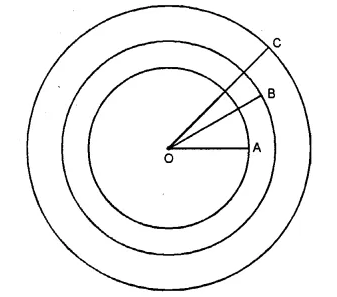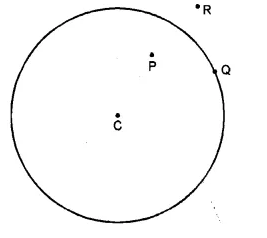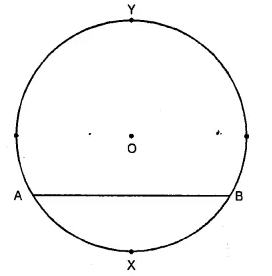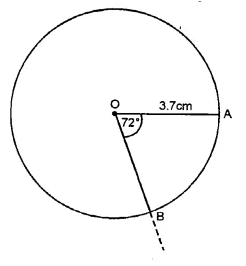# RS Aggarwal Class 6 Solutions Chapter 18 Circles Ex 18

## RS Aggarwal Class 6 Solutions Chapter 18 Circles Ex 18

These Solutions are part of RS Aggarwal Solutions Class 6. Here we have given RS Aggarwal Solutions Class 6 Chapter 18 Circles Ex 18

Question 1.
Solution:
Method :Take a point O on the paper as shown in the figure. With the help of the rular, open out compasses in such a way that the distance between the metal point and pencil point is 4 cm. Take the compasses in the same position and put its metal point at O and draw the circle.
Remove the compasses and again open out the compasses in such a way that the distance between the metal point and pencil point is 5.3 cm. Taking O as the centre, draw another circle. Again remove the compasses and similarly draw the third circle with radius 6.2 cm. Then the required circles are as shown in the figure which have radius OA = 4 cm., OB = 5.3 cm. and OC = 6.2 cm.

Question 2.
Solution:
Method : Take a point C on the paper. With the help of the rular, open out the compasses in such a way that the distance between its metal point and pencil point is 4.5 cm. Take the compasses in the same position and put its metal point at C and draw the circle. Mark points P, Q and R as shown in the figure as required.Question 3.
Solution:
Method : Take a point O on the paper. With the help of the rular, open out the compasses in such a way that the distance between the metal point and pencil point is 4 cm. Take the compasses in the same position and put the metal point at O and draw the circle.Take A and B any points on the circle and join AB. ThenAB is the chord of the circle. Mark points X and Y on the circle as shown. Then arc AXB and arc AYB are the required minor and major arcs respectively.

Question 4.
Solution:
(i) False
(ii) True
(iii) False
(iv) False
(v) True.

Question 5.
Solution:
Steps of construction :
(i) With centre O and radius 3.7 cm, draw a circle.
(ii) Take a point A on the circumference of the circle.
(iii) Join OA.
(iv) At O, draw another radius OB such that ∠AOB = 72° with the help of protractor. Then sector AOB is the required one.Question 6.
Solution:
(i) > (ii) < (iii) > (iv) >. Ans.

Question 7.
Solution:
(i) Passes through
(ii) at the centre, on the circle
(iii) chord
(iv) arc
(v) sector.

Hope given RS Aggarwal Solutions Class 6 Chapter 18 Circles Ex 18 are helpful to complete your math homework.

If you have any doubts, please comment below. Learn Insta try to provide online math tutoring for you.